A Simplified Dynamic Model for Constant-Force Compression Spring

Ikechukwu Celestine UGWUOKE

Department of Mechanical Engineering, Federal University of Technology, Minna, Niger State, Nigeria.

E-mail(s):ugwuokeikechukwu@yahoo.com

Abstract

A simplified dynamic model for the constant-force compression spring (CFCS) based on the pseudo-rigid-body model (PRBM) is presented including the basic formulations which takes care of the moment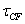due to coulomb friction in the pin joints of the CFCS, and the moment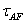due to axial force effects in the rigid links of the CFCS. The CFCS is a slider mechanism which consists of rigid links incorporating pin joints and a small-length flexural pivot which connects to a slider. Clearly the results with the inclusion ofandto the dynamic model, shows that the static terms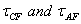have very great significance on the dynamic model of the CFCS.

Keyword

Constant-force compression spring, Axial force effects, Coulomb friction, Pseudo-rigid-body model.

Introduction

With the emerging applications of compliant mechanisms, there is the need to develop a systematic formulation for the design and analysis of compliant mechanisms. Although existing methods such as the finite element method (FEM), elliptic integrals method, and chain algorithm method are widely available, there remain challenges in the computational model of compliant mechanisms (CMs). Many of these existing models are either inadequate to capture the geometric nonlinearity or too complicated that they cannot serve as a basis for compliant mechanism (CM) design and simulation. Based on the principle of dynamic equivalence, a simplified dynamic model for the constant-force compression spring (CFCS) is developed using the pseudo-rigid-body (PRB) modeling technique. The pseudo-rigid-body model (PRBM) is used to simplify the analysis and design of CMs. It is used to unify CM and rigid-body mechanism theory by providing a method of modeling the nonlinear deflection of flexible beams. This method of modeling allows well-known rigid-body analysis methods to be used in the analysis of CMs (Salamon, 1989). The PRBM provides an easy way to model the complex, nonlinear deflections of many CMs (Howell, 2001). The model approximates the force-deflection characteristics of a compliant segment using two or more rigid segments joined by pin joints, with torsional springs at the joints modeling the segment’s stiffness. The usefulness of the PRBM in allowing accurate analysis and synthesis of mechanism motion and energy storage characteristics has been abundantly demonstrated (Opdahl et al., 1998; Derderian et al., 1996; Howell and Midha, 1996; Lyon et al., 1997; Jensen et al., 1997; Mattlach and Midha, 1996). While the model is very useful for the analysis of CMs, its true power lies in the capability it gives for designing original CMs (Jensen et al.’ 1997). The PRBM correlates the synthesis of CMs and the wealth of knowledge available in rigid-body mechanism design. CFCSs can be defined as mechanisms that produce a constant output force for a large range of input displacements. Such mechanisms are important in applications with varying displacements, but requiring a constant resultant output force. The mechanism is typically displacement driven, the input is a displacement at the slider and the output is a force. Unlike a linear spring where the force increases as the displacement increases, the reaction force for a CFCS remains constant for various displacements.

Simplified Dynamic Model Development

Figure 1 shows the CFCS and its PRBM. As shown in the figure, the CFCS consists of rigid links incorporating pin joints and a small-length flexural pivot which connects to a slider. The mechanism is converted to its rigid-body counterpart by using the PRBM rule for small-length flexural pivots. The most straight forward alteration is that the small-length flexural pivot becomes a pin and torsional spring combination, centered at the middle of the flexible segment. The torsional spring constant K for small-length flexural pivots is given by

K=EI/L                                                                                                            (1)

Where,

I is the moment of inertia of the cross section of the flexible segment

E is the modulus of elasticity of the flexible segment

L is the length of the flexible segment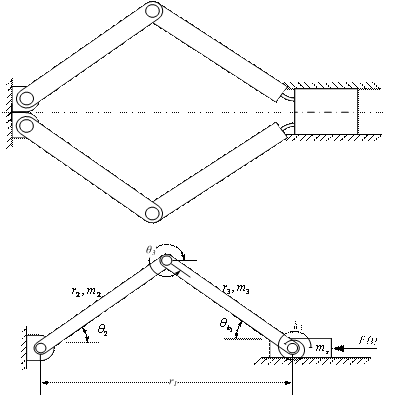Figure 1: CFCS and Its pseudo-rigid-body model

Formulation of the Dynamic Equation using Hamilton’s Principle

The dynamic equation of motion for the CFCS can be systematically derived using Hamilton’s principle, where the following variational form holds: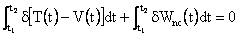(2)

Equation (2), which is generally known as Hamilton’s variational statement of dynamics, shows that the sum of the time-variations of the difference in kinetic and potential energies and the work done by the nonconservative forces over any time interval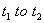equals zero. It is of interest to note that Hamilton’s equation can also be applied to statics problems. In this case, the kinetic energy term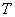vanishes, and the remaining terms in the integrands of equation (2) are invariant with time; thus, equation (2) reduces to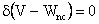(3)

Equation (3) is the well known principle of minimum potential energy, so widely used in static analysis. For most mechanical or structural systems, the kinetic energy can be expressed in terms of the generalized coordinates and their first time derivatives, and the potential energy can be expressed in terms of the generalized coordinates alone (Clough and Penzien, 2003). In addition, the virtual work which is performed by the nonconservative forces as they act through the virtual displacements caused by an arbitrary set of variations in the generalized coordinates can be expressed as a linear function of those variations. In mathematical terms the above three statements are expressed in the form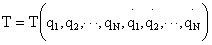(4)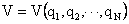(5)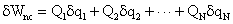(6)

where the coefficients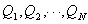are the generalized forcing functions corresponding to the coordinates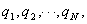respectively. Substituting equation (4), (5), and (6) into (2) and completing the variation of the first term gives(7)

Integrating the velocity-dependent terms in equation (7) by paths leads to(8)

The first term on the right hand side of equation (8) is equal to zero for each coordinate since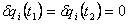is the basic condition imposed upon the variations. Substituting equation (8) into equation (7) gives, after rearranging terms,(9)

where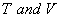are the kinetic and potential energy of the CFCS respectively. Since all variations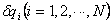are arbitrary, equation (9) can be satisfied in general only when the term in bracket vanishes.(10)

Equations (10) are the well known Lagrange’s equations of motion, which have found widespread application in various fields of science and engineering. It should be noted that Lagrange’s equations are a direct result of applying Hamilton’s variational principle, under the specific condition that the energy and work terms can be expressed in terms of the generalized coordinates, and of their time derivatives and variations. Thus Lagrange’s equations are applicable to all systems which satisfy these restrictions, and they may be nonlinear as well as linear (Clough and Penzien, 1995). Following the standard procedure of Hamilton’s principle, the total kinetic energy equation for the CFCS is given as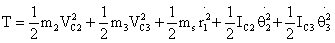(11)

where,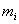= mass of links 2 and 3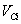= velocity of the center of mass of links 2 and 3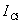= mass moment of inertia of links 2 and 3 about the center of mass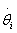= angular velocity of links 2 and 3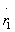= velocity of the slider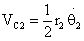(12)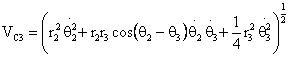(13)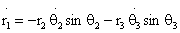(14)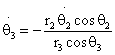(15)

The first three terms of the kinetic energy expression represent the translational energy of the system, and the last two represent the rotational energy. The mass moments of inertia of links 2 and 3 about the center of mass is given by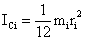(16)

For the CFCS, the potential energy equation is given as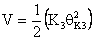(17)

where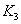is the torsional spring constant and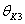is the relative deflections of the torsional spring given by the following expression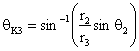(18)

Expanding equation (10) and simplifying, the dynamic equation for the system becomes(19)

To obtain the generalized forcing function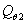, the virtual work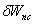must be evaluated. This is the work performed by all nonconservative forces acting on or within the flexural member while an arbitrary set of virtual displacements is applied to the system. The nonconservative forces may include dissipative forces proportional to the angular and linear velocities. For the CFCS, the generalized forcing functiontherefore consists of a moment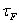due directly to the force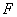acting on the slider, momentdue to coulomb friction in the pin joints of the CFCS, and momentdue to axial force effects in the rigid links of the CFCS. In mathematical terms, the generalized forcing functionis given by the expression below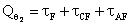(20)

Coulomb friction results from two dry or lubricated surfaces rubbing together. It is generally considered to be independent of velocity magnitude but has a different, larger value when velocity is zero (static friction) than when there is relative motion between the parts (dynamic friction). Though more elaborate expressions for the coulomb friction termare possible, the following simple relation gives sound results: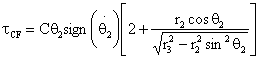(21)

where C is the coulomb friction coefficient(22)

The value of the torqueis chosen using experimental data but may be approximated using the expression giving in equation (22). Torqueis transformed to mechanism’s output force F using the power relationship given as: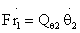(23)(24)

where,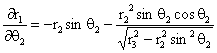(25)

Equations (19)–(25) represent the simplified dynamic model of the CFCS. Note that the equation of motion was derived from the PRBM of the CFCS, rather than the actual CFCS. What is important in the derivation of the dynamic model is not the method chosen to arrive at the simplified dynamic model, but the fact that the method was applied to the PRBM simplification of the CFCS.

Results and Discussion

All the necessary parameters of the CFCS used in the simulation are given in table 1. Using these parameters, the simulation results obtained are illustrated in the following figures. Figure 2 shows a comparison of the force predicted by the static portion of the dynamic model (i.e. with velocities and accelerations set to zero, with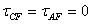), with that predicted by existing CFCS theory, essentially an application of the principle of virtual work on the PRBM of the CFCS. As shown in the figure, both plots match perfectly which is a confirmation that the static portion of the dynamic model is correct. Figure 3 shows the force predicted by the static portion of the dynamic model with inclusion of. As shown in the figure,have large effect on the performance of the CFCS.

Table 1: CFCS parameters used for simulation

 Parameters Parameter Values r2 85 mm r3 95 mm m2 0.025kg m3 0.028 kg ms 0.087kg b 25.4 mm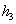0.38 mm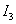1.1615 x 10-13 m4 E 207 Gpa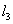9.5 mm2.5308 Nm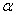0.045 rad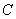0.02

Figure 5, 6, and 7 shows the mean force plot, the median force plot and the peak-to-peak force magnitude difference plot of the dynamic model as a function of frequency respectively. Each frequency assumes a sinusoidal position input as shown in Figure 4 with amplitude equal to 40% mechanism deflection. Notice that the curve in the peak-to-peak force plots for the dynamic model with the inclusion ofandfirst curves down, before it starts to increase. This shows the range of frequencies over which a CFCS exhibits better constant force behaviour. Figure 8, 9, 10, and 11 shows the plots of the force predicted by the dynamic model with, and without the inclusion of the static termsfor different input frequencies. Clearly as shown in the figures, the results with the inclusion of, shows that the static termshave very great significance on the dynamic model. Figure 12 shows the plot of the percent constant-force (PCF) as a function of time. The PCF is very important because it measures the amount of variation between the minimum and maximum output force of the CFCS model. As shown in the figure, the maximum value of the PCF for the CFCS model is 87.2%.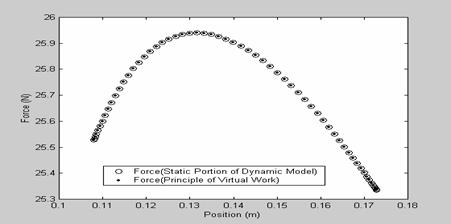Figure 2: Force predicted by the static portion of the dynamic model

with that predicted by the principle of virtual work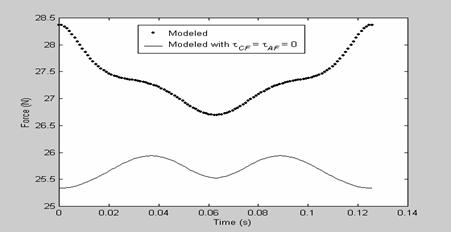Figure 3: Force predicted by the static portion of the dynamic model

with inclusion of tCF and tAF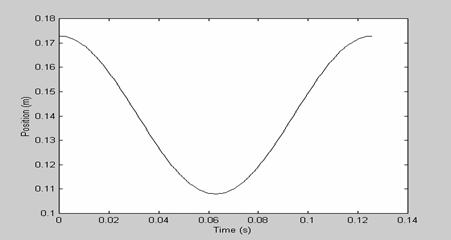Figure 4: Position plot representing the sinusoidal input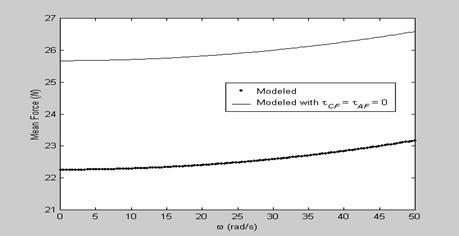Figure 5: The mean force plot as a function of frequency for the CFCS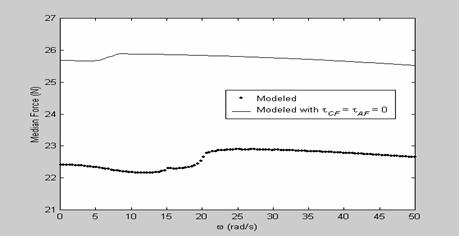Figure 6: The median force plot as a function of frequency for the CFCS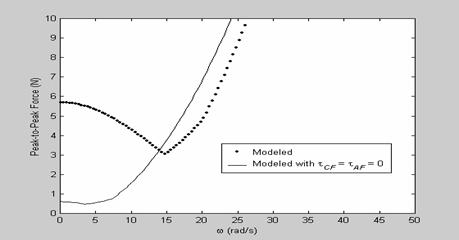Figure 7: The peak-to-peak force difference plot as a function of frequency for the CFCS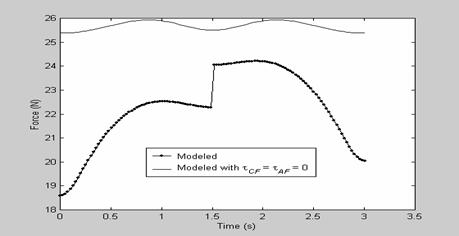Figure 8: Predicted force for sinusoidal input of ω = 2.09 rad/s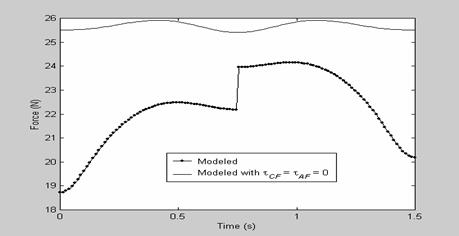Figure 9: Predicted force for sinusoidal input of ω = 4.19 rad/s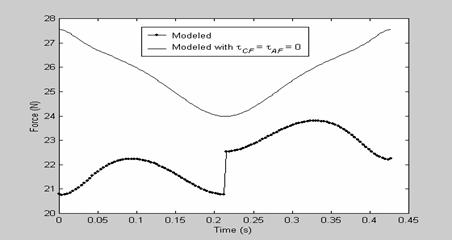Figure 10: Predicted force for sinusoidal input of ω = 14.7 rad/s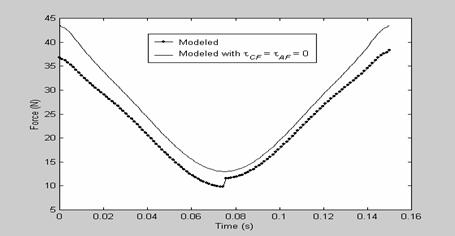Figure 11: Predicted force for sinusoidal input of ω = 41.89 rad/s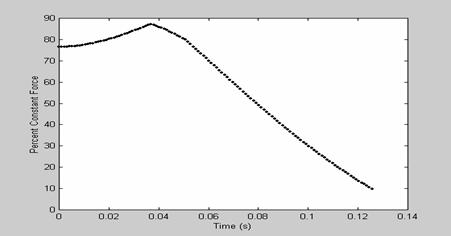Figure 12: Percent constant-force as a function of time

Conclusion

The PRBM is a method of analysis that allows large deformations to be modeled using rigid-body kinematics. In light of the simplicity the PRBM affords, a simplified dynamic model for the CFCS based on the PRBM is presented which also includes basic formulations which take care of the axial force effects in the rigid links of the CFCS and the effect due to Coulomb friction in the pin joints of the CFCS. Although several methods for modeling CFCS exist, less attention has been paid to dynamic analysis. A very interesting aspect of the dynamic model is that it presents a very simplified and accurate mathematical expression which helps in the easy determination of the momentdue to coulomb friction in the pin joint of the CFCS, and the momentdue to axial force effects in the rigid links of the CFCS. Clearly the results with the inclusion ofandto the dynamic model, shows that the static termshave very great significance on the dynamic model of the CFCS.

References

1.      Derderian, J. M., “The Pseudo-Rigid-Body Model Concept and its Application to Micro Compliant Mechanisms”, M. S. Thesis, Brigham Young University, Provo, Utah. 1996.

2.      Jensen, B. D., Howell, L. L., Gunyan, D. B., and Salmon, L. G., “The Design and Analysis of Compliant MEMS Using the Pseudo-Rigid-Body Model”, Microelectromechanical Systems (MEMS) 1997, 1997 ASME International Mechanical Engineering Congress and Exposition, Nov. 16-21, 1997, Dallas, TX, DSC-Vol. 62, pp. 119-126, 1997.

3.      Lyon, S. M., Evans, M. S., Erickson, P. A., and Howell, L. L, “Dynamic Response of Compliant Mechanisms Using the Pseudo-Rigid-Body Model”, Proceedings of the 1997 ASME Design Engineering Technical Conferences, DETC97/VIB-4177, 1997.

4.      Howell, L. L., and Midha, A., ‘‘A Loop-Closure Theory for the Analysis and Synthesis of Compliant Mechanisms,’’ ASME Journal of Mechanical Design, 118, No. 1, pp. 121–125, 1996.

5.      Howell, L. L., “Compliant Mechanisms”, John Wiley & Sons, New York, 2001.

6.      Opdahl, P. G., Jensen, B. D., and Howell, L. L., “An Investigation into Compliant Bistable Mechanisms”, Proc. of 1998 ASME Design Engineering Technical Conferences, DETC98/MECH-5914, 1998.

7.      Mettlach, G. A., and Midha, A., “Using Burmester Theory in the Design of Compliant Mechanisms”, Proceedings of the 1996 ASME Design Engineering Technical Conferences, 96-DETC/MECH-1181, 1996.

8.      Salamon, B. A., “Mechanical Advantage Aspects in Compliant Mechanisms Design”, M.S. Thesis, Purdue University, West Lafayette, Indiana, 1989.

9.      Clough, R.W.; and Penzien, J., “Dynamics of Structures”, Third Edition, Computer and Structures, Inc., University Ave. Berkeley, CA 94704, USA, 2003.